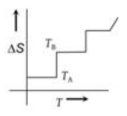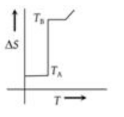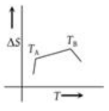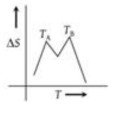A sink, that is the system where heat is rejected, is essential for the conversion of heat into work. From which law the above inference follows?

(1) zeroth

(2) First

(3) Second

(4) Third

Concept Questions :-

Second law of thermodynamics
High Yielding Test Series + Question Bank - NEET 2020

Difficulty Level:

If for a given substance melting point is ${T}_{B}$ and freezing point is ${T}_{A}$, then correct variation shown by graph between entropy change and temperature is

1.2.3.4.Concept Questions :-

Second law of thermodynamics

Difficulty Level:

A Carnot engine having an efficiency of $\mathrm{\eta }=\frac{1}{10}$ as
heat engine is used as a refrigerator. If the work
done on the system is 10 J, then the amount of
energy absorbed from the sink at lower temperature
is
1. 100 J

2. 99 J

3. 90 J

4. 1 J

Concept Questions :-

Second law of thermodynamics